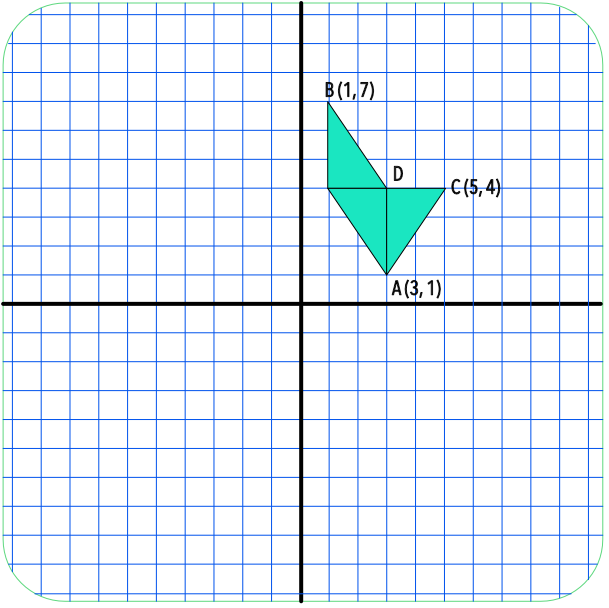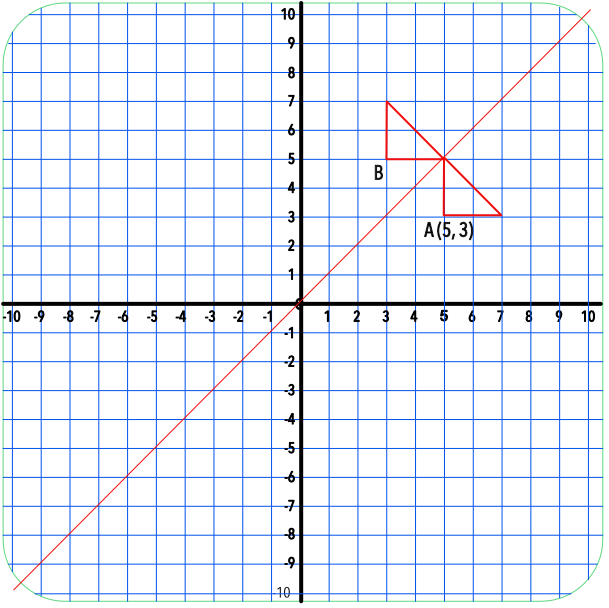Solving Geometry Problems on Axes

# Solving Geometry Problems on Axes

GCSE(F),

Problems involving coordinates on axes need to be related to the (x, y) coordinates of the axes. Draw or plot any given lines or coordinates. Answers should be given as coordinates (unless otherwise specified).

## Examples

1. Three congruent triangles are shown below. What are the coordinates of point D?The triangles are congruent and are therefore all the same size.

The height of two triangles is given by the y-coordinate of B minus the y-coordinate of A

7 - 1 = 6

The height of one triangle = 6 ÷ 2 = 3

Point D is immediately above point A, so the x-coordinate is unchanged.

Point D = (3, 1 + 3) = (3, 4)

2. A triangle has a right angle at point A. the triangle is reflected in the line x=y. What are the coordinates of point B?